### Reasoning Practice Test IBPS Clerk Seating Arrangement Coded Inequalities

Eight members of a club are sitting around a circular table, viz Z, Y, X, W, V, U, T and S, but not
necessarily in the same order. Four of them are not facing the centre. W is facing the center and is third to the right of Z, who is third to the right of U, who is facing the center. X-is fourth to the left of T, S is third to the right of V, who is not a neighbour of T. V sits second to the right of X. Y is on the immediate right of T.

1) What is S's position with respect to Y?
a) 2nd to the left.
b) 3rd to the left.
c) 2nd to the right.
d) Either (a) or (c).
e) None.

2) Who is second to the left of V?
a) W
b) X
c) Y
d) T
e) None of these

3) Which of the following pairs are neighbours ofT?
a) W, Y
b) U, S
c) Z, W
d) Can't be determined
e) None of these

4) Four of the following five are alike in a certain way and so form a group. Which is the one that does not belong to that group?
a) W
b) Y
c) V
d) Z
e) U

5) Which of the following statements is/are true?
a) Z is not facing the center.
b) Y is second to the right of W.
c) T is facing the center.
d) Both (a) and (b) are true.
e) None of these.

Directions: In the following questions, the symbols \$,@,%, © and # are used with the following meanings as illustrated below:
'P©Q' means 'P' is greater than 'Q'.
'P%Q' means 'P' is smaller than 'Q'.
'P@Q' means 'P' is either greater than or equal 'Q'.
'P\$Q' means 'P' is either smaller than or equal to 'Q'.
'P#Q' means 'P' is equal to 'Q'.
A) If only conclusion I is true.
B) If only conclusion II is true.
C) If either conclusion I or II is true.
D) If neither conclusion I nor II is true.
E) If both conclusions I and II are true

6) Statements: M @ R, R ©F, F#L
Conclusions: I. R@L II.M@L

7) Statements: T % J, J @ V, V # W
Conclusions: I. T©W II. W@T

8) Statements: J @ D, D\$ L, L#N
Conclusions: I. J # L II. J \$ L

9) Statements: R \$ M, M%H,H\$F
Conclusions: I. R % F II. M\$F

10) Statements: K \$ H, H % I, I © F
Conclusions: I. K \$ I II.H % F

1) C
2) D
3) A
4) B
5) E
6) D
7) C
8) D
9) A
10) D

Explanation:(1-5)

(6-7)
6) (D) M ≥ R>F=L. So, R>L. Hence, conclusion I is not true. Even, the Conclusion II is not true.
7) (C) T < J ≥ V= W Either I or II follows.
8) (D) J ≥ D ≤ L = N Both the conclusions are not true
9) (A) R ≤ M < H ≤ F. Hence, R< F. Conclusion I is true. As M< F conclusion II is not true.
10) (D) K ≤ H < I > F. As K< I, conclusion I is not true. H and F can't be compared. Hence, conclusion II is not true

1.Answer of 8 should be C not D. If I am wrong then please explain?

1.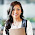No ans is neither nor
1st and 2nd Conclusions are not related...
see rules from

2.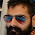8 ka ans c Hona chAye

3.No Vivek, Answer is D itself check the rules again... If you still having doubt then post it here I will clarify it.

4.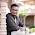5.Hi DJA, i rhave a doubt regarding first question. If we interchange the position of X and T we get different answer but i think the answer is right.

6.There is no need to consider X and T to answer 1st question right...
And why do you want to interchange them anyway?

7.Thank u for yo reply and questions. Its really helpful

8.you are welcome

2.Then, 7th conclusion also not related. Please explain further for the 7th question. It is better if u explain the either concept further.

1.question got slight mistake corrected now

2.answer 7 should be D it cannot be C as T=V=W as relationship cannot be estb b/w T&W or W&T

3.How did you get T = V = W?

3.plz explain how this arrangment is made

4.Plz explain me this arrangement when some people facing centre and some not , then how to arrange clock and antivlock wise direction? Plz explain me ... Plz reply soon plzzz

1.Hi Pooja,
Thanks for commenting your doubt...
Remember these two points
1. For every puzzle or seating arrangement there will be a staring point but that may not be in the starting lines of question, some at the last lines or middle of given question.

2. You have to take a guess or hunch at some point based on the given question to continue. If you won't take chance like that you can't continue because most of the questions are framed like that.

I will post a photo of explanation here in an hour please refer to that...

2.Hi again Pooja,
Explanation was posted above please check it...

3.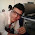please send the explanation .... i have
a doubt how you can decide v is center faced ?

4.What if i choose vice versa position of t and x as you assumed their position ?

5.Excellent explanation......keep it up

1.Thank you Abhi

6.simple one...

7.Simplest Question.....

8.explaination not visible

1.Click on the photo you can see it then...

9.10.how did you fix the position of w?

1.Please see the explanation pic

11.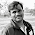I did all correct except 7th one....nw pretty clear !!! Thank u ......

1.Keep Up the good work Shivaraj

12.Still I am confused regarding direction,pls explain the arrangements

13.how you are decididng who is facing centre and not.there is only one clue for that? pls explain,

1.I'm not deciding who is facing center. If you read the question clearly till "U Who is facing center" you will get the correct positions try it again

14.In question no.10,

Conclusion 1 "K \$ I" is true.

Conclusion 2 "H % F" is can't be compare.

So, the answer must be option A. If only conclusion I is true.

1.Hi Dinesh,
How can you say 1st conclusion is true
As per question we get
K < I is correction but in conclusion they asked K ≤ I
How can these two are same?

15.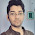Thanks for the trick

1.you are welcome Avinash

16.Am not able to solve quest 7

1.Why, what is the problem?

17.How u can say Z facing inside

18.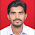S and V's direction are in doubt...if S is not facing towards centre then how can be it 2nd to the right of Y (as given in 1 of the questions answer)
Plzz clear the doubt...

19.how is the ans of 5 E? Please explain.

20.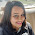how is ans of 6 D plz explain.

1.Hi Anjali,
Please see the explanation given below the Answers you will find the solution...

21.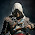Hi there,
in the first puzzle the question says that four are not facing centre which means there can be two three or five or six facing the centre..How did you assume that 4 are facing inwards and four are facing outwards.....and how did the direction of Y or S was calculated

1.Hi Ashray,
Don't understand the given question in too complicated way. In these type of questions if they mentioned 4 are not facing center just take that remaining are facing in opposite direction ok. I hope you got the point.

22.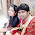am getting different answer for this but that also satisfies all the condition...

23.i get different answer but it also satisfy all the conditions given in the question....